OMB Home        White House WebsitePresident's Budget
Management
Information &
Regulatory Affairs
Legislative Information
Agency Information

September xx10, 2001

Performing Reestimates with the Revised Credit Subsidy Calculator

The Credit Subsidy Calculator (CSC) software, released November 26, 1999, has been revised to support the calculation of basket-of-zeros reestimates, as well as similar maturity reestimates.  Revision 1c now supports reestimates of subsidies originally calculated under the “basket-of-zeros” method (cohorts from FY 2001 onward).  Revision 1c of the CSC continues to calculate reestimates for the FY 1992 through FY 2000 cohorts (“similar maturity” cohorts) using methods similar to the methods used by the former DOS-based Credit Subsidy Model.  Therefore, in most cases, the results for these cohorts should be identical to the results obtained using the DOS-based software.

For the FY 2002 Budget, agencies could choose to perform reestimates using the DOS-based Credit Subsidy Model or the Windows-based Credit Subsidy Calculator.  Beginning with the FY 2003 Budget, agencies are required to calculate reestimates of all cohorts using the Credit Subsidy Calculator.

This document discusses the steps involved in calculating reestimates using the revised CSC.

A.  Revise the Cash Flows for the Revised CSC

To calculate reestimates using the revised CSC, the agency must make a few changes to the cash flow spreadsheet:

1.               Insert “Purpose” Line: Insert a line with this keyword in the descriptive information section of the cash flows.  Enter the word “Reestimate” in the cell to the immediate right of the “Purpose” keyword.  (See row 5 in the examples below.)

2.               Insert “Maturity of Loans” Line: This keyword is required for the FY 1992 through FY 2000 cohorts only.  For these cohorts, insert a line with the “Maturity of Loans” keyword in the descriptive information section of the cash flows to apply to all cohorts.  If the named range contains a mixture of similar maturity and basket-of-zeros cohorts, then the CSC will ignore the keyword line for the basket-of-zeros cohorts.  To apply the keyword to a specific similar maturity cohort, insert the keyword line in the cash flow data section for that cohort.  (For more information on placement of this keyword line, see Appendix B at the end of this document.)  The “maturity of loans” is the average length, in number of years, of the direct or guaranteed loans in the cohort.  Enter the loan maturity, in number of years, in the cell to the immediate right of the “Maturity of Loans” keyword.  (See row 6 in the similar maturity example below.)

3.         Insert “Reestimate Discount Rate” Line: Insert a line with this keyword in the descriptive information section of the cash flows to apply to all cohorts.  To apply the keyword to a specific cohort, insert the keyword line in the cash flow data section for that cohort. (For more information on placement of this keyword line, see Appendix B at the end of this document.)  In the cell to the immediate right of the keyword, insert one of the following values: “use 90 percent”; “use all”; or the numeric discount rate (the weighted average rate for similar maturity cohorts or the single effective rate for basket-of-zeros cohorts).  These options are discussed further under “Select Weighted-Average Discount Rate Calculation Method” below and in Appendix A to this document.  (See row 7 in the similar maturity example below and row 6 in the basket-of-zeros example below.)

4.         Enter “Cohort” Line: Enter a line with this keyword immediately above the “Obligations” or “Commitments” line.  (If direct loan cash flows do not include the “Obligations” keyword line, enter this line directly above the “Disbursements” keyword line.)  (See row 10 in the similar maturity example below and row 9 in the basket-of-zeros example below.)

Cash Flow Example, Similar Maturity Cohort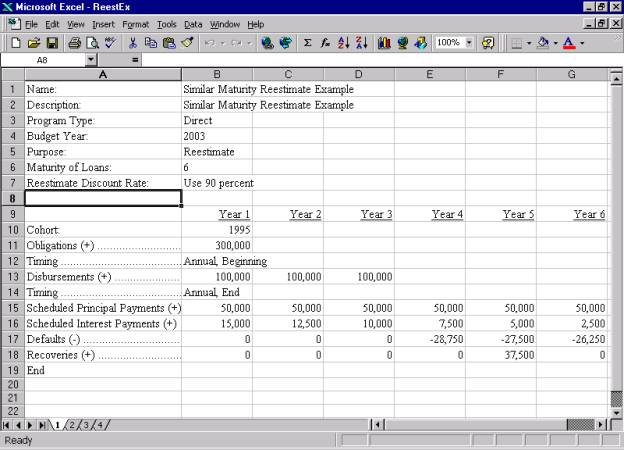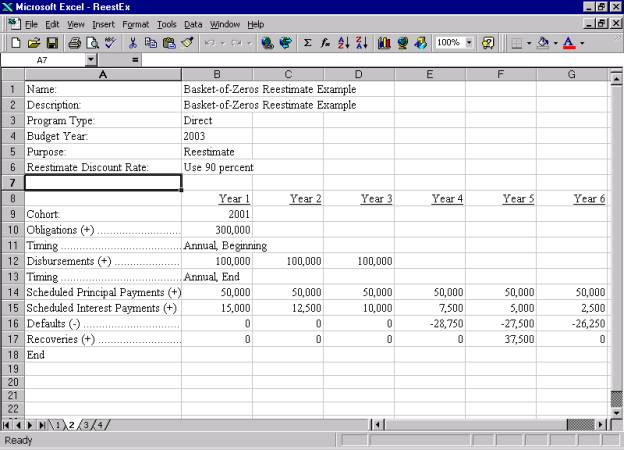B.  Select Weighted-Average Discount Rate Calculation Method

The revised CSC allows the agency to choose how the disbursement-weighted average discount rate is calculated.  The agency may select the “Use 90 percent” method, the “Use all” method, or may enter a numeric discount rate into the cash flow spreadsheet.  Each of these options is detailed below.

For the FY 1992 to FY 2000 cohorts, the CSC uses a single disbursement weighted average rate to discount the cash flows.  The CSC calculates this rate from the similar maturity rates that are built into the software.  Therefore, the agency does not need to enter any discount rates into the cash flow spreadsheet.

For the FY 2001 and future cohorts, the CSC uses a disbursement weighted average yield curve (unless a single effective rate is supplied by the agency, as described below).  Like the similar maturity rates, the yield curve rates are built into the CSC.  However, after the cohort is fully disbursed, the agency will calculate the final single effective rate for the cohort.  The agency will then need to include the final single effective rate in the reestimate cash flows for all subsequent reestimates.

Indicate the interest rate calculation method or the appropriate numeric interest rate on the “Reestimate Discount Rate” keyword line.  The possible values for this keyword line include:

·       “Use 90 Percent”: When this method is chosen, the CSC uses the original budget assumption discount rates until the cohort is at least 90 percent disbursed.  Once the cohort is at least 90 percent disbursed, the CSC uses the actual average interest rates for those disbursement years.  (See Appendix A for further details.)

·       When to use this value: Use this value if the agency performs interest rate reestimates once for each cohort, after the cohort is at least 90 percent disbursed, in accordance with OMB Circular A-11, Section 85.5.  If the agency uses the 90-percent disbursement rule, use this value whether the cohort has not yet reached, is at, or is past the 90 percent disbursement point.

·       For similar maturity cohorts: Continue to use this value for the full life of the direct or guaranteed loans.

·       For basket-of-zeros cohorts: Use this value until the direct or guaranteed loans are fully disbursed (90 percent under the use 90 percent method, 100 percent under the use all method) and the final single effective rate is calculated for the cohorts.  Once the final single effective rate is calculated, specify that number in all future reestimate cash flows.

·       “Use All”: When this method is chosen, the CSC uses all actual average interest rates available for disbursement years.  The CSC uses the original budget assumption discount rates for years for which actual rates are not yet available.  (See Appendix A for further details.)

·       When to use this value: Use this value if the agency performs interest rate reestimates for all actual interest rates, regardless of the proportion of the cohort that has disbursed to date.

·       For similar maturity cohorts: Continue to use this value for the full life of the direct or guaranteed loans.

·       For basket-of-zeros cohorts: Use this value until the direct or guaranteed loans are fully disbursed (90 percent under the use 90 percent method, 100 percent under the use all method) and the final single effective rate is calculated for the cohorts.  Once the final single effective rate is calculated, specify that number in all future reestimate cash flows.

·       Numeric Interest Rate: For similar maturity cohorts, enter the cohort disbursement-weighted average discount rate.  For basket-of-zeros cohorts, enter the single effective rate.  Enter the interest rate as a number to two decimal places and without the percent sign.  For example, enter 5.25 percent as “5.25.”

·       When to use a numeric interest rate:

·       For similar maturity cohorts: Enter the agency’s own numeric value for analytic exercises or when the preceding two options do not fit the agency’s needs. (Note that the use of a non-standard weighted-average interest rate for budget purposes must be approved in advance by OMB.)

·       For basket-of-zeros cohorts: Use this value once the cohort of direct or guaranteed loans are fully disbursed (90 percent under the use 90 percent method, 100 percent under the use all method) and the final single effective rate has been calculated.  (See the example below.  In this case, the reestimate is being performed for the FY 2005 Budget.  The loans were fully disbursed following the end of FY 2003.  The final single effective rate was calculated in FY 2004, and is specified in the FY 2005 and all subsequent reestimates for this cohort.)  This option may also be used for analytic exercises or when the preceding two options do not fit the agency’s needs.  (Note that the use of a non-standard weighted-average interest rate for budget purposes, other than use of the final single effective rate, must be approved in advance by OMB.)

Cash Flow Example, Basket-of-Zeros Cohort Reestimate, After Calculation of Final Single Effective Rate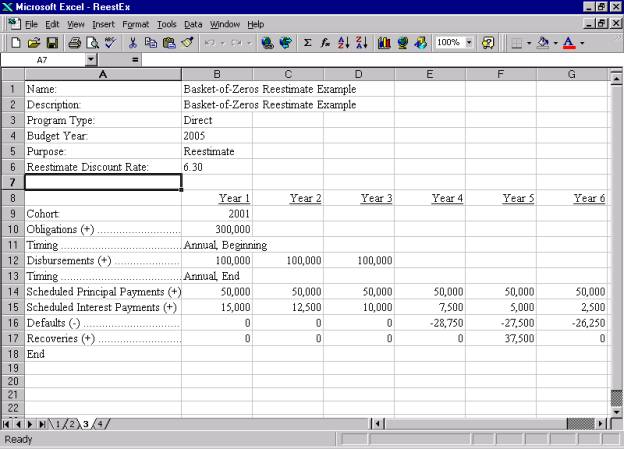C.  Calculate the Interest Rate Reestimate

If the agency is not calculating an interest rate reestimate for the cohort this year, skip to step D, “Calculate the Technical Reestimate.”

Interest rate reestimates account for the difference between the interest rate assumption used to calculate the subsidy rate for budget execution and the actual average interest rate(s) prevailing during the year(s) of disbursement.  This difference includes the effects of actual discount rates, as well as actual borrower interest rates in cases where the borrower’s interest rate is tied to some benchmark rate.  In addition, the borrower’s interest rate may also affect other cash flow items, such as the level of total principal and interest defaults expected.

Interest rate reestimates are calculated using the original subsidy cash flows updated only for actual interest rates and their effects.

To perform an interest rate reestimate using the revised CSC:

1.     Make sure that the original cash flows include the changes described in step A and B:

a.      Verify that there is a “Purpose” keyword line that specifies that the purpose is “reestimates.”

b.     For FY 1992 through FY 2000 cohorts, verify that there is a “Maturity of Loans” keyword line that specifies the average maturity of the loans in the cohort.

c.      Verify that there is a “Reestimate Discount Rate” keyword line that specifies, “use 90 percent,” “use all,” or a single effective rate or weighted-average interest rate, as appropriate.  (Note that the use of a non-standard weighted-average interest rate must be approved in advance by OMB.)

d.     Verify that there is a “Cohort” keyword line that specifies the cohort fiscal year of the direct loans or loan guarantees being reestimated.

2.     Open the revised CSC (if the software is not already open).

3.     Open the cash flow file using, “File, Open,” in the CSC.

4.     Calculate the subsidy (see the example “Subsidy Output Display 1” below).  This is the interest rate reestimate subsidy rate.

5.     The interest rate reestimate is the difference between the interest rate reestimate subsidy rate obtained in step 4 and the original subsidy rate used for budget execution.

Note that the subsidy display provides the “weighted average rate” for similar maturity cohorts and the “single effective rate” for basket-of-zeros cohorts.

Subsidy Output Display 1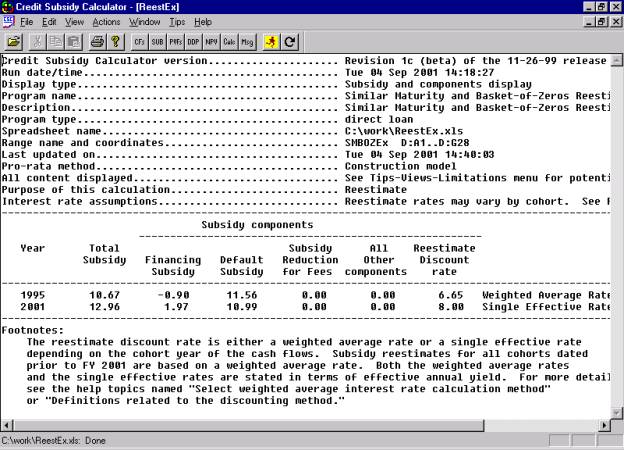The present value factor display presents further detail on the weighted-average rate.  (See the example present value factor display below.)  If the cash flow spreadsheet indicates the “use 90 percent” or the “use all” approach, the present value factor display will detail the calculation of the weighted-average interest rate. The display presents: the disbursement year; the level of disbursements for each year; and the applicable discount rate or yield curve for that year (based on the average interest rate calculation method selected).  The display also presents the resulting weighted-average discount rate or single effective rate.  If the cash flow spreadsheet specifies a weighted-average interest rate or single effective rate, the display will indicate that rate.

Present Value Factor Display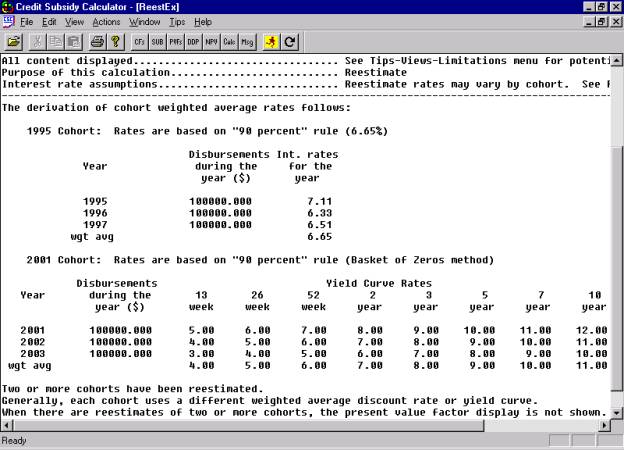D.  Calculate the Technical Reestimate

Technical reestimates account for changes in technical assumptions that underlie the cash flow estimates, such as assumptions about disbursements, defaults, delinquencies, repayment patterns, recoveries, fees, and other cash flow elements.  Technical reestimates are calculated using the cash flows updated for any available actual data and revised projections for future years

To perform a technical reestimate using the revised CSC:

1.     Make sure that the revised cash flows include the changes described in steps A and B:

a.      Verify that there is a “Purpose” keyword line that specifies that the purpose is “reestimates.”

b.     For FY 1992 through FY 2000 cohorts, verify that there is a “Maturity of Loans” keyword line that specifies the average maturity of the loans in the cohort.

c.      Verify that there is a “Reestimate Discount Rate” keyword line that specifies, “use 90 percent,” “use all,” or a single effective rate or weighted-average interest rate, as appropriate.  (Note that the use of a non-standard weighted-average interest rate must be approved in advance by OMB.)  This value must be identical to the value used for any interest rate reestimates performed for the cohort in the current year.  The value must also be consistent with the approach used for the cohort in any previous interest rate reestimates or expected to be used in any future interest rate reestimates.  If the correct value is entered on this line, the CSC will calculate the technical reestimate consistent with any interest rate reestimates performed for the cohort in the current, previous, or future years.

d.         Verify that there is a “Cohort” keyword line that specifies the cohort fiscal year of the direct loans or loan guarantees being reestimated.

2.     Open the revised CSC (if the software is not already open).

3.     Open the cash flow file using, “File, Open,” in the CSC.

4.     Calculate the subsidy (see “Subsidy Output Display 2” below).  This is the technical reestimate subsidy rate.

5.     The technical reestimate is the difference between the technical reestimate subsidy rate obtained in step 4 and the original subsidy rate used for budget execution.

6.     For basket-of-zeros cohorts that have become fully disbursed (90percent under the use 90 percent method, 100 percent under the use all method) in the previous fiscal year, record the single effective rate.  This single effective rate will serve as the final single effective rate for the cohort, and must be used in all future technical reestimates.

Subsidy Output Display 2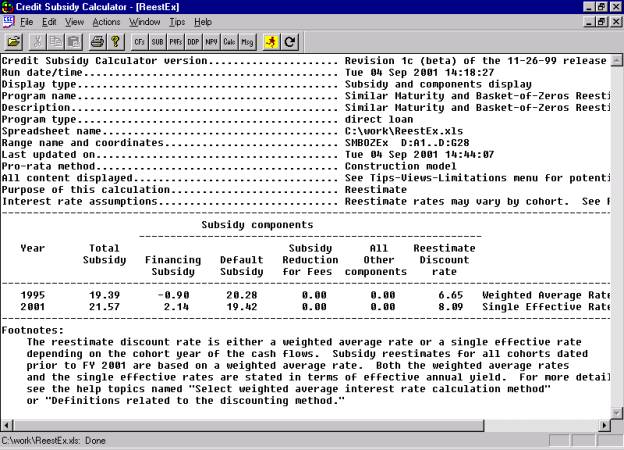Note that the subsidy display provides the “weighted average rate” for similar maturity cohorts and the “single effective rate” for basket-of-zeros cohorts.

E.  Complete the Reestimate Process

Enter the interest rate reestimate (if any) and the technical reestimate in the appropriate sections of the Reestimate Calculator.  The Reestimate Calculator and accompanying instructions (“Using the Reestimate Calculator to Calculate Reestimates and Interest on the Reestimate”) are available on the Federal Credit Support Page, /omb/credit.  For further detail on reestimates, see OMB Circular A-11, Section 85.5.

## Appendix A: The CSC Methods for Calculating the Weighted-Average Interest Rate

The CSC  supports three ways to provide a discount rate (using the “Reestimate Discount Rate” keyword line in the credit subsidy cash flows):

·       Use the “use 90 percent” rule and have a disbursement-weighted average rate or yield curve calculated automatically.  For similar maturity (FY 1992 – 2000) cohorts the CSC calculates a single disbursement average discount rate.  For basket-of-zeros (FY 2001 and future) cohorts, the CSC calculates a disbursement weighted average yield curve.  The results will be shown in the display for present value factors (see the example in section C of this document).

·       Use the “use all” rule and have a disbursement-weighted average rate or yield curve calculated automatically.  For similar maturity  cohorts the CSC calculates a single disbursement average discount rate.  For basket-of-zeros cohorts after the CSC calculates a disbursement weighted average yield curve.  The results will be shown in the display for present value factors.

·       Specify a specific rate.  For similar maturity  cohorts this option may be used for budget purposes only with prior OMB approval.  For basket-of-zeros cohorts, use this value once the cohort of direct or guaranteed loans is fully disbursed and the final single effective rate has been calculated.

This section present details on how these choices work.

The “use 90 percent” rule specifies that the reestimate discount rate or yield curve is the disbursement-weighted average of actual rates calculated when the cohort is 90 percent disbursed.  If there are too few actual rates for the 90 percent rule, then the budget assumptions will be used.

Here are some examples:

# Similar maturity cohort examples

For a 1995 cohort that fully disburses in that year, the 1995 actual average annual rate would be used as the reestimate discount rate.

For a 1994 cohort that disburses 80 percent in 1994, 11 percent in 1995, and 9 percent in 1996, the weighted-average rate would be computed as:

At the end of 1994:

1994 Budget assumption rate

At the end of 1995 and for all subsequent years:

[ ( 0.8  ·  1994 rate ) + ( 0.11  ·  1995 rate ) ] / 0.91

For a 2000 cohort that disburses 50 percent in 2000, 30 percent in 2001, and 20 percent in 2002, no interest rate reestimate would be done until the actual rates for 2002 become available.  The budget assumptions for the 2000 cohort will be used until the actual rates for 2002 become available.

For a 2001 cohort that fully disburses in that year, the actual full yield curve would be used to calculate a single effective rate for the reestimate.  For a 2001 cohort that disburses over multiple years, in following years, as new actual yield curves become available, the CSC will use those rates to calculate a disbursement-average yield curve.

As an example, assume that actual rates exist through FY 2003 and that the FY 2001 cohort disburses 80 percent in FY 2001, 11 percent in FY 2002, and 9 percent in FY 2003, the disbursement weighted average yield curve would be calculated as follows:

Hypothetical actual rates:

Year    13w     26w     52w     2yr       3yr       5yr       7yr       10yr     30yr

2001    5.00     6.00     7.00     8.00     9.00     10.00   11.00   12.00   13.00

2002    5.50     6.50     7.50     8.50     9.50     10.50   11.50   12.50   13.50

2003   4.00     5.00     6.00     7.00     8.00     9.00     10.00   11.00   12.00

Since the cohort was 91 percent disbursed in 2002, each rate in FY 2001 is multiplied by (80/91), each rate in FY 2002 is multiplied by (11/91) with the sum of the two being the disbursement-weighted average for that point on the yield curve.  The FY 2003 rates are ignored since the cohort was at least 90 percent disbursed by FY 2002:

Year    13w     26w     52w     2yr       3yr       5yr       7yr       10yr     30yr

2001    4.40     5.27     6.15     7.03     7.91     8.79     9.67     10.55   11.43

2002    0.66     0.79     0.91     1.03     1.15     1.27     1.39     1.51     1.63

total     5.06     6.06     7.06     8.06     9.06     10.06   11.06   12.06   13.06

The CSC then uses this new disbursement-weighted yield curve to calculate the reestimated subsidy and the reestimate single effective rate.

The “use all” rule specifies that the reestimate discount rate or yield curve is the disbursement-weighted average of any and all actual rates, when available, and budget assumptions otherwise.

Here are some examples:

# Similar maturity cohort examples

For a 1995 cohort that fully disburses in that year, the 1995 average annual rate would be used as the reestimate discount rate

For a 1994 cohort that disburses 80 percent in 1994, 11 percent in 1995, and 9 percent in 1996, the weighted-average rate would be computed as follows:

At the end of 1994:

[ ( 0.8  ·  1994 rate ) + ( 0.2  ·  1994 budget assumption rate)] / 1.0

At the end of 1995:

[ ( 0.8  ·  1994 rate ) + ( 0.11  ·  1995 rate )

+ ( 0.09  ·  1994 budget assumption rate)] / 1.0

At the end of 1996 and for all subsequent years:

[ ( 0.8  ·  1994 rate ) + ( 0.11  ·  1995 rate )

+ ( 0.09  ·  1996 rate)] / 1.0

For a 2001 cohort that fully disburses in 2001, the CSC will use the actual FY 2001 yield curve to calculate the reestimate and the reestimate single effective rate.  In following years, as new actual yield curves become available, the CSC will use those rates to calculate a disbursement-average yield curve.

As an example, assume that the FY 2001 cohort disburses 80 percent in FY 2001, 11 percent in FY 2002, and 9 percent in FY 2003.  For FY 2001, FY 2002, and FY 2003 the disbursement-weighted average yield curve would be calculated as follows:

At the end of FY 2001 with hypothetical actual rates for FY 2001 being:

13w     26w     52w     2yr       3yr       5yr       7yr       10yr     30yr

2001    5.00     6.00     7.00     8.00     9.00     10.00   11.00   12.00   13.00

and FY 2001 budget assumptions of:

13w     26w     52w     2yr       3yr       5yr       7yr       10yr     30yr

2001    5.48     5.63     5.71     5.81     5.88     5.97     6.03     6.10     6.27

The disbursement-weighted yield curve would be calculated by multiplying each FY 2001 actual rate by (80/100), each FY 2001 Budget rate by (20/100) (the weight of the remaining disbursements) and summing the results.  The CSC would use this yield curve to generate the reestimate single effective rate for FY 2001.

At the end of FY 2002 with hypothetical actual rates for FY 2002 being:

13w     26w     52w     2yr       3yr       5yr       7yr       10yr     30yr

2002    5.50     6.50     7.50     8.50     9.50     10.50   11.50   12.50   13.50

The disbursement-weighted yield curve would be calculated by multiplying each FY 2001 actual rate by (80/100), each FY 2002 actual rate by (11/100), each FY 2001 Budget rate by (9/100) (the weight of the remaining disbursements) and summing the results.  The CSC would use this yield curve to generate the reestimate single effective rate for FY 2002.

At the end of FY 2003 with hypothetical actual rates for FY 2003 being:

13w     26w     52w     2yr       3yr       5yr       7yr       10yr     30yr

2003   4.00     5.00     6.00     7.00     8.00     9.00     10.00   11.00   12.00

The disbursement-weighted yield curve would be calculated by multiplying each FY 2001 actual rate by (80/100), each FY 2002 actual by (11/100), each FY 2001 actual rate by (9/100), and summing the results.  The CSC would use this yield curve to generate the reestimate single effective rate for FY 2003 and beyond.

If the cash flow spreadsheet specifies a numeric interest rate, then the CSC will use the specified rate.  The revised CSC will use only the interest rate in the cell to the immediate right of the “Reestimate Discount Rate” keyword line.  For basket-of-zeros cohorts this value should be used once the cohort is fully disbursed (90 percent disbursed under the use 90 percent method, 100 percent disbursed under the use all method) and the final single effective rate has been calculated.

Appendix B: Including Multiple Cohorts in a Reestimate Cash Flow

The revised CSC allows agencies to include multiple cohorts in a reestimate cash flow.  The CSC also allows the agency to specify different loan maturities and weighted-average discount rate calculation methods for each cohort.

Indicating the Cohort

When multiple cohorts are included in a cash flow, each new cohort must begin with a new “Cohort” keyword line that specifies the cohort fiscal year.  For example, the cash flow below indicates on row 8 that the first cohort is fiscal year 1995 and indicates on row 20 that the second cohort is fiscal year 2001.

Indicating the Maturity of Loans (FY 1992-FY 2000 Cohorts Only)

The revised CSC provides two ways to indicate the maturity of loans:

·       Specify the maturity of loans in all cohorts: To specify the maturity of the loans in all cohorts, include the “Maturity of Loans” keyword in the upper descriptive information section of the cash flows.  For an example, see row 6 of the similar maturity cash flow example in section A of this document.

·       Specify the maturity of loans for each cohort: To specify the maturity of loans separately for each cohort, include the “Maturity of Loans” keyword in the cash flow section for each cohort.  For an example, see the cash flow spreadsheet below.  This option is useful for cases in which the loans of different cohorts have different average maturities.  For example, in the cash flow below, the 1995 cohort has a 6-year average maturity (row 9).  If other similar maturity cohorts were included in this cash flow, the maturity could be similarly specified.  (Note that the FY 2001 cohort, a basket-of-zeros cohort, does not include the “Maturity of Loans” keyword.

If the “Maturity of Loans” keyword appears in the descriptive information section, then the CSC will use that maturity value for all cohorts in the cash flow unless a specific maturity is presented for that cohort.

Indicating the Weighted-Average Interest Rate Calculation Method

Similar to “maturity of loans,” the revised CSC provides two ways to indicate the weighted-average interest rate calculation method (or a specific weighted average rate or single effective rate):

·       Specify the calculation method (or numeric interest rate) for all cohorts: To specify the calculation method to use for all cohorts, include the “Reestimate Discount Rate” keyword in the upper descriptive information section of the cash flows.  For an example, see row 7 of the similar maturity cash flow example or row 6 of the basket-of-zeros example in section A of this document.

·       Specify the calculation method (or weighted-average interest rate) for each cohort: To specify the calculation method separately for each cohort, include the “Reestimate Discount Rate” keyword in the cash flow section for each cohort.  For an example, see the cash flow below (rows 10 and 21).

If the “Reestimate Discount Rate” keyword appears in the descriptive information section, then the CSC will use that calculation method (or numeric interest rate) for all cohorts in the cash flow unless a specific calculation method (or numeric interest rate) is presented for that cohort.

(Note that, as a result of screen size limitations, rows 1 through 6 do not appear in the example cash flow below.  These rows contain the descriptive information for the cash flow.)

Appendix B Cash Flow Example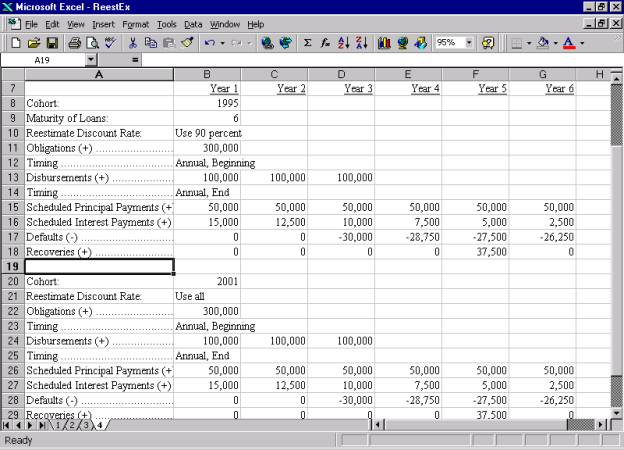Credit Subsidy Calculator Results

When multiple cohorts are included in a cash flow, the CSC will calculate a separate reestimated subsidy rate for each cohort.  For examples, see the subsidy output display examples in sections C and D of this document.

The CSC also calculates a separate weighted average rate or single effective rate for each cohort.  For an example, see the present value factor example in section C of this document.

 For information on cash flow spreadsheets, see the working paper, “How to Organize Cashflow Estimates in a Spreadsheet,” available in the CSC Help files and on the Federal Credit Support Page, /omb/credit.

 Alternatively, the purpose may be specified as reestimates in the CSC software, under “Actions, Preferences, General Preferences.”

 The “single effective rate” is the single discount rate that will produce the same subsidy rate as the full yield curve of discount rates.  For cohorts that disburse over more than one year, the single effective rate will represent the disbursement weighted average of the yield curves for each disbursement year.  For more information on single effective rate, see the working paper, “How the single effective rate is calculated,” available on the Federal Credit Support Page, /omb/credit.

 Under the use 90 percent method, “fully disbursed” is defined as at least 90 percent disbursed.  Under the use all method, “fully disbursed” as defined as 100 percent disbursed, net of cancellations.

 Instructions for using the CSC are provided in the document, “Getting Started,” available in the CSC Help files.

 Instructions for using the CSC are provided in the document, “Getting Started,” available in the CSC Help files.

 Under the use 90 percent method, “fully disbursed” is defined as at least 90 percent disbursed.  Under the use all method, “fully disbursed” as defined as 100 percent disbursed, net of cancellations.